# Reasoning MCQ - 14

## 30 Questions MCQ Test Reasoning Aptitude for Competitive Examinations | Reasoning MCQ - 14

Description
Attempt Reasoning MCQ - 14 | 30 questions in 30 minutes | Mock test for LR preparation | Free important questions MCQ to study Reasoning Aptitude for Competitive Examinations for LR Exam | Download free PDF with solutions
QUESTION: 1

### Directions : Study the following information carefully and answer the questions given below: Amartya Sen, Liu Xiaobo, Gerhard Domagk, Albert Fert, Andre Geim and Hideki Yukaw are six Nobel laureates, who belong to six different countries viz–India, China, Germany, France, Russia and Japan but not necessarily in the same order, each one has won the prize in different fields, viz Physics, Chemistry, Economics, Medicine, Peace and Literature but not necessarily in the same order. They are sitting around a circular table facing the centre. The persons who have won the prize for Medicine, Economics and Peace are neither from Japan nor from India. The persons who are from France and China have won the prizes neither for Medicine nor for Literature. Amartya Sen is neither from Japan nor sits on the immediate left of the person who won the prize for Chemistry. The only person who is between Andre Geim and Hideki Yukaw has won the prize for Physics. The person who is on the left side of the person from Japan has not won the prize for Literature. Albert Fert has won the prize for Chemistry and is from China. He is facing th  e person who has won the prize for Physics. One who has won the prize for Medicine is sitting opposite the person from Russia, while the person from China is on the left of the person who has won the prize for peace. One who has won the prize for Literature is on the immediate right of the person from Japan but on the left of the person who has won the prize for Economics. Gerhard Domagk has not won the prize for Economics and Hideki Yukaw has not won the prize for Medicine. Q. Who is Second To the left of Gerhard Domagk?

Solution: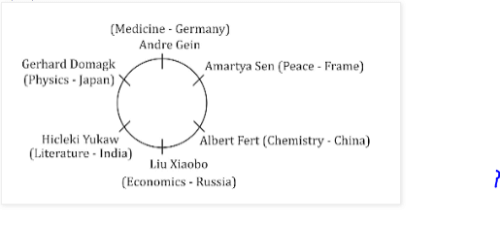QUESTION: 2

### Directions : Study the following information carefully and answer the questions given below: Amartya Sen, Liu Xiaobo, Gerhard Domagk, Albert Fert, Andre Geim and Hideki Yukaw are six Nobel laureates, who belong to six different countries viz–India, China, Germany, France, Russia and Japan but not necessarily in the same order, each one has won the prize in different fields, viz Physics, Chemistry, Economics, Medicine, Peace and Literature but not necessarily in the same order. They are sitting around a circular table facing the centre. The persons who have won the prize for Medicine, Economics and Peace are neither from Japan nor from India. The persons who are from France and China have won the prizes neither for Medicine nor for Literature. Amartya Sen is neither from Japan nor sits on the immediate left of the person who won the prize for Chemistry. The only person who is between Andre Geim and Hideki Yukaw has won the prize for Physics. The person who is on the left side of the person from Japan has not won the prize for Literature. Albert Fert has won the prize for Chemistry and is from China. He is facing th  e person who has won the prize for Physics. One who has won the prize for Medicine is sitting opposite the person from Russia, while the person from China is on the left of the person who has won the prize for peace. One who has won the prize for Literature is on the immediate right of the person from Japan but on the left of the person who has won the prize for Economics. Gerhard Domagk has not won the prize for Economics and Hideki Yukaw has not won the prize for Medicine. Q. Who likes Economics?

Solution: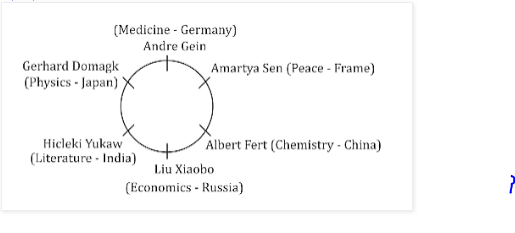QUESTION: 3

### Directions : Study the following information carefully and answer the questions given below: Amartya Sen, Liu Xiaobo, Gerhard Domagk, Albert Fert, Andre Geim and Hideki Yukaw are six Nobel laureates, who belong to six different countries viz–India, China, Germany, France, Russia and Japan but not necessarily in the same order, each one has won the prize in different fields, viz Physics, Chemistry, Economics, Medicine, Peace and Literature but not necessarily in the same order. They are sitting around a circular table facing the centre. The persons who have won the prize for Medicine, Economics and Peace are neither from Japan nor from India. The persons who are from France and China have won the prizes neither for Medicine nor for Literature. Amartya Sen is neither from Japan nor sits on the immediate left of the person who won the prize for Chemistry. The only person who is between Andre Geim and Hideki Yukaw has won the prize for Physics. The person who is on the left side of the person from Japan has not won the prize for Literature. Albert Fert has won the prize for Chemistry and is from China. He is facing th  e person who has won the prize for Physics. One who has won the prize for Medicine is sitting opposite the person from Russia, while the person from China is on the left of the person who has won the prize for peace. One who has won the prize for Literature is on the immediate right of the person from Japan but on the left of the person who has won the prize for Economics. Gerhard Domagk has not won the prize for Economics and Hideki Yukaw has not won the prize for Medicine. Q. Who is the third to the left of one who likes Medicine?

Solution:QUESTION: 4

Directions : Study the following information carefully and answer the questions given below:Amartya Sen, Liu Xiaobo, Gerhard Domagk, Albert Fert, Andre Geim and Hideki Yukaw are six Nobel laureates, who belong to six different countries viz–India, China, Germany, France, Russia and Japan but not necessarily in the same order, each one has won the prize in different fields, viz Physics, Chemistry, Economics, Medicine, Peace and Literature but not necessarily in the same order. They are sitting around a circular table facing the centre. The persons who have won the prize for Medicine, Economics and Peace are neither from Japan nor from India. The persons who are from France and China have won the prizes neither for Medicine nor for Literature. Amartya Sen is neither from Japan nor sits on the immediate left of the person who won the prize for Chemistry. The only person who is between Andre Geim and Hideki Yukaw has won the prize for Physics. The person who is on the left side of the person from Japan has not won the prize for Literature. Albert Fert has won the prize for Chemistry and is from China. He is facing th  e person who has won the prize for Physics. One who has won the prize for Medicine is sitting opposite the person from Russia, while the person from China is on the left of the person who has won the prize for peace. One who has won the prize for Literature is on the immediate right of the person from Japan but on the left of the person who has won the prize for Economics. Gerhard Domagk has not won the prize for Economics and Hideki Yukaw has not won the prize for Medicine.

Q.

Who among the following wins prize for Physics?

Solution:QUESTION: 5

Directions : Study the following information carefully and answer the questions given below:

Amartya Sen, Liu Xiaobo, Gerhard Domagk, Albert Fert, Andre Geim and Hideki Yukaw are six Nobel laureates, who belong to six different countries viz–India, China, Germany, France, Russia and Japan but not necessarily in the same order, each one has won the prize in different fields, viz Physics, Chemistry, Economics, Medicine, Peace and Literature but not necessarily in the same order. They are sitting around a circular table facing the centre. The persons who have won the prize for Medicine, Economics and Peace are neither from Japan nor from India. The persons who are from France and China have won the prizes neither for Medicine nor for Literature. Amartya Sen is neither from Japan nor sits on the immediate left of the person who won the prize for Chemistry. The only person who is between Andre Geim and Hideki Yukaw has won the prize for Physics. The person who is on the left side of the person from Japan has not won the prize for Literature. Albert Fert has won the prize for Chemistry and is from China. He is facing th  e person who has won the prize for Physics. One who has won the prize for Medicine is sitting opposite the person from Russia, while the person from China is on the left of the person who has won the prize for peace. One who has won the prize for Literature is on the immediate right of the person from Japan but on the left of the person who has won the prize for Economics. Gerhard Domagk has not won the prize for Economics and Hideki Yukaw has not won the prize for Medicine.

Q.

Which of the following combinations is true?

Solution:QUESTION: 6

Directions : Study the following information carefully and answer the questions given below:

Amartya Sen, Liu Xiaobo, Gerhard Domagk, Albert Fert, Andre Geim and Hideki Yukaw are six Nobel laureates, who belong to six different countries viz–India, China, Germany, France, Russia and Japan but not necessarily in the same order, each one has won the prize in different fields, viz Physics, Chemistry, Economics, Medicine, Peace and Literature but not necessarily in the same order. They are sitting around a circular table facing the centre. The persons who have won the prize for Medicine, Economics and Peace are neither from Japan nor from India. The persons who are from France and China have won the prizes neither for Medicine nor for Literature. Amartya Sen is neither from Japan nor sits on the immediate left of the person who won the prize for Chemistry. The only person who is between Andre Geim and Hideki Yukaw has won the prize for Physics. The person who is on the left side of the person from Japan has not won the prize for Literature. Albert Fert has won the prize for Chemistry and is from China. He is facing th  e person who has won the prize for Physics. One who has won the prize for Medicine is sitting opposite the person from Russia, while the person from China is on the left of the person who has won the prize for peace. One who has won the prize for Literature is on the immediate right of the person from Japan but on the left of the person who has won the prize for Economics. Gerhard Domagk has not won the prize for Economics and Hideki Yukaw has not won the prize for Medicine.

Q.

Hideki Yukaw is from which of the following country?

Solution:QUESTION: 7

Directions : Directions: In the following questions, two Conclusion is given and five statements are given as a), b), c), d) and e. From this you have to take the statements to be true even if they seem to be at variance with commonly known facts and then decide which of the given statement logically follows.

Q.

Conclusions:

All Donald being Micky is a possibility.

All Tom being Jerry is a possibility.

Solution: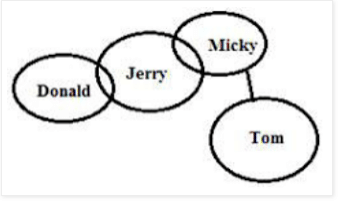QUESTION: 8

Directions : Directions: In the following questions, two Conclusion is given and five statements are given as a), b), c), d) and e. From this you have to take the statements to be true even if they seem to be at variance with commonly known facts and then decide which of the given statement logically follows.

Q.

Conclusions:

Some Table is plastic.

Some plastic are bench

Solution: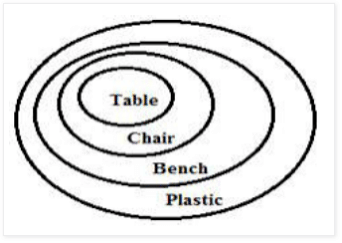QUESTION: 9

Directions : Directions: In the following questions, two Conclusion is given and five statements are given as a), b), c), d) and e. From this you have to take the statements to be true even if they seem to be at variance with commonly known facts and then decide which of the given statement logically follows.

Q.

Conclusions:

Some dates are day.

Some years are day.

Solution: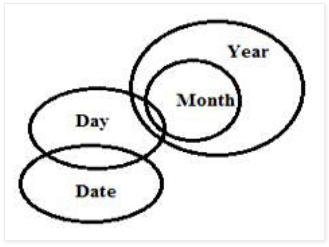QUESTION: 10

Directions : Directions: In the following questions, two Conclusion is given and five statements are given as a), b), c), d) and e. From this you have to take the statements to be true even if they seem to be at variance with commonly known facts and then decide which of the given statement logically follows.

Q.

Conclusions:

Some teachers are not student.

Some lessons are classroom.

Solution: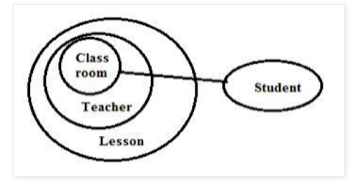QUESTION: 11

Directions : Directions: In the following questions, two Conclusion is given and five statements are given as a), b), c), d) and e. From this you have to take the statements to be true even if they seem to be at variance with commonly known facts and then decide which of the given statement logically follows.

Q.

Conclusions:

All windows being cot is a possibility.

Some doors are not pillow.

Solution: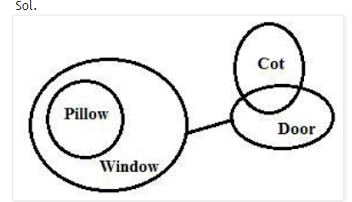QUESTION: 12

Directions : Consider the following statements and the questions that follow. We will analyse this problem in detail after that. In a certain code language

"login Mentors and check" is coded as "\$4e %4g #2n @6t"

"reads article study there" is coded as "@6i #4u \$4e %4a"

"comment about yours doubt" is coded as "\$4u @4o %6m #4u"

"get clear exams enjoy" is coded as "@4e #4a \$4j %2e"

Q.

What is the code for exams?

Solution:
QUESTION: 13

Directions : Consider the following statements and the questions that follow. We will analyse this problem in detail after that. In a certain code language

"login Mentors and check" is coded as "\$4e %4g #2n @6t"

"reads article study there" is coded as "@6i #4u \$4e %4a"

"comment about yours doubt" is coded as "\$4u @4o %6m #4u"

"get clear exams enjoy" is coded as "@4e #4a \$4j %2e"

Q.

What is the code for study?

Solution:
QUESTION: 14

Directions : Consider the following statements and the questions that follow. We will analyse this problem in detail after that. In a certain code language

"login Mentors and check" is coded as "\$4e %4g #2n @6t"

"reads article study there" is coded as "@6i #4u \$4e %4a"

"comment about yours doubt" is coded as "\$4u @4o %6m #4u"

"get clear exams enjoy" is coded as "@4e #4a \$4j %2e"

Q.

What is the code for about?

Solution:
QUESTION: 15

Directions : Consider the following statements and the questions that follow. We will analyse this problem in detail after that. In a certain code language

"login Mentors and check" is coded as "\$4e %4g #2n @6t"

"reads article study there" is coded as "@6i #4u \$4e %4a"

"comment about yours doubt" is coded as "\$4u @4o %6m #4u"

"get clear exams enjoy" is coded as "@4e #4a \$4j %2e"

Q.

If we code "study Mentors clear exams" as per the above coding method, what will be the code?

Solution:
QUESTION: 16

Directions : In the following questions, the symbols \$, @, £, • and # are used with the following meanings as illustrated below:

‘A \$ B' means A is neither greater nor smaller than B

‘A @ B' means A is neither greater than nor equal to B

`A £ B' means A is neither smaller than nor equal to B

‘A • B' means A is not smaller than B

‘A # B' means A is not greater than B

In each of the following questions, assuming the given statements to be true, find out which of the two conclusions I and II given below them is/are definitely true. Give answer

Q.

Statements: W • P,  P £ G,  G @ I,  I # N Conclusions: I. I £ P  II. N # W

Solution:
QUESTION: 17

Directions : In the following questions, the symbols \$, @, £, • and # are used with the following meanings as illustrated below:

‘A \$ B' means A is neither greater nor smaller than B

‘A @ B' means A is neither greater than nor equal to B

`A £ B' means A is neither smaller than nor equal to B

‘A • B' means A is not smaller than B

‘A # B' means A is not greater than B

In each of the following questions, assuming the given statements to be true, find out which of the two conclusions I and II given below them is/are definitely true. Give answer

Q.

Statements: U @ D,  D \$ E,  E £ Y,  Y • W

Conclusions: I. W @ E  II. D £ W

Solution:
QUESTION: 18

Directions : In the following questions, the symbols \$, @, £, • and # are used with the following meanings as illustrated below:

‘A \$ B' means A is neither greater nor smaller than B

‘A @ B' means A is neither greater than nor equal to B

`A £ B' means A is neither smaller than nor equal to B

‘A • B' means A is not smaller than B

‘A # B' means A is not greater than B

In each of the following questions, assuming the given statements to be true, find out which of the two conclusions I and II given below them is/are definitely true. Give answer

Q.

Statements: Z £ N,  N # K,  K \$ M,  M @ R

Conclusions: I. M \$ N  II. M £  N

Solution:
QUESTION: 19

Directions : In the following questions, the symbols \$, @, £, • and # are used with the following meanings as illustrated below:

‘A \$ B' means A is neither greater nor smaller than B

‘A @ B' means A is neither greater than nor equal to B

`A £ B' means A is neither smaller than nor equal to B

‘A • B' means A is not smaller than B

‘A # B' means A is not greater than B

In each of the following questions, assuming the given statements to be true, find out which of the two conclusions I and II given below them is/are definitely true. Give answer

Q.

Statements: V • D,  D £ T,  K \$ T,  K # F

Conclusions: I. D £  K  II. T • F

Solution:
QUESTION: 20

Directions : In the following questions, the symbols \$, @, £, • and # are used with the following meanings as illustrated below:

‘A \$ B' means A is neither greater nor smaller than B

‘A @ B' means A is neither greater than nor equal to B

`A £ B' means A is neither smaller than nor equal to B

‘A • B' means A is not smaller than B

‘A # B' means A is not greater than B

In each of the following questions, assuming the given statements to be true, find out which of the two conclusions I and II given below them is/are definitely true. Give answer

Q.

Statements: S \$ Q,  Q @ B,  B • K,  K # W

Conclusions: I. K # S  II. S @ W

Solution:
QUESTION: 21

Directions : In the following questions the symbols @, +, ©, \$, ∆  and ? are used with the following meaning:

P ∆ Q means P is not equal to Q.

P @ Q means P is greater than Q.

P + Q means P is smaller than Q.

P © Q means P is either greater than or equal to Q.

P \$ Q means P is either smaller than or equal to Q.

P ? Q means P is equal to Q.

Now in each of the following questions assuming the given statements to be true, ﬁnd which of the two conclusions I and ll given below them is/are deﬁnitely true. Give answer.

Solution:
QUESTION: 22

Directions : In the following questions the symbols @, +, ©, \$, ∆  and ? are used with the following meaning:

P ∆ Q means P is not equal to Q.

P @ Q means P is greater than Q.

P + Q means P is smaller than Q.

P © Q means P is either greater than or equal to Q.

P \$ Q means P is either smaller than or equal to Q.

P ? Q means P is equal to Q.

Now in each of the following questions assuming the given statements to be true, ﬁnd which of the two conclusions I and ll given below them is/are deﬁnitely true. Give answer

Q.

Statements:K © M,     M ∆ R,    R ? T

Conclusions: I. K © T  II. M ? T

Solution:
QUESTION: 23

Directions : In the following questions the symbols @, +, ©, \$, ∆  and ? are used with the following meaning:

P ∆ Q means P is not equal to Q.

P @ Q means P is greater than Q.

P + Q means P is smaller than Q.

P © Q means P is either greater than or equal to Q.

P \$ Q means P is either smaller than or equal to Q.

P ? Q means P is equal to Q.

Now in each of the following questions assuming the given statements to be true, ﬁnd which of the two conclusions I and ll given below them is/are deﬁnitely true. Give answer

Q.

Statements: B+D,      D@N,    N\$H

Solution:
QUESTION: 24

Directions : In the following questions the symbols @, +, ©, \$, ∆  and ? are used with the following meaning:

P ∆ Q means P is not equal to Q.

P @ Q means P is greater than Q.

P + Q means P is smaller than Q.

P © Q means P is either greater than or equal to Q.

P \$ Q means P is either smaller than or equal to Q.

P ? Q means P is equal to Q.

Now in each of the following questions assuming the given statements to be true, ﬁnd which of the two conclusions I and ll given below them is/are deﬁnitely true. Give answer

Q.

Conclusions: I. M@N  II.M?N

Solution:
QUESTION: 25

Directions : In the following questions the symbols @, +, ©, \$, ∆  and ? are used with the following meaning:

P ∆ Q means P is not equal to Q.

P @ Q means P is greater than Q.

P + Q means P is smaller than Q.

P © Q means P is either greater than or equal to Q.

P \$ Q means P is either smaller than or equal to Q.

P ? Q means P is equal to Q.

Now in each of the following questions assuming the given statements to be true, ﬁnd which of the two conclusions I and ll given below them is/are deﬁnitely true. Give answer

Q.

Statements: T\$M,     M?Q,     Q+R

Conclusions: I. Q @ T  II.Q ? T

Solution:
QUESTION: 26

Directions : In the following questions the symbols @, +, ©, \$, ∆  and ? are used with the following meaning:

P ∆ Q means P is not equal to Q.

P @ Q means P is greater than Q.

P + Q means P is smaller than Q.

P © Q means P is either greater than or equal to Q.

P \$ Q means P is either smaller than or equal to Q.

P ? Q means P is equal to Q.

Now in each of the following questions assuming the given statements to be true, ﬁnd which of the two conclusions I and ll given below them is/are deﬁnitely true. Give answer

Q.

Statements: D @ B,   B\$T,    T + M

Solution:
QUESTION: 27

Directions:  In the following questions, the symbols @, \$, %, # and © are used with the following meanings illustrated—

(i) ‘X @ Y’ means ‘X is not smaller than Y’.

(ii) ‘X \$ Y’ means ‘X is not greater than Y’.

(iii) ‘X % Y’ means ‘X is neither smaller than nor equal to Y’.

(iv) ‘X # Y’ means ‘X is neither greater than nor equal to Y’.

(v) ‘X © Y’ means ‘X is neither smaller than nor greater than Y’.

In each of the following questions assuming the given statements to be true, find out which of the two conclusions I and II given below them is/are definitely true. Give answer

Q.

Statements:  P \$ T,  T @ L,   U % L

Conclusions: I. P @ L II. U©L

Solution:
QUESTION: 28

Directions:  In the following questions, the symbols @, \$, %, # and © are used with the following meanings illustrated—

(i) ‘X @ Y’ means ‘X is not smaller than Y’.

(ii) ‘X \$ Y’ means ‘X is not greater than Y’.

(iii) ‘X % Y’ means ‘X is neither smaller than nor equal to Y’.

(iv) ‘X # Y’ means ‘X is neither greater than nor equal to Y’.

(v) ‘X © Y’ means ‘X is neither smaller than nor greater than Y’.

In each of the following questions assuming the given statements to be true, find out which of the two conclusions I and II given below them is/are definitely true. Give answer

Q.

Solution:
QUESTION: 29

Directions:  In the following questions, the symbols @, \$, %, # and © are used with the following meanings illustrated—

(i) ‘X @ Y’ means ‘X is not smaller than Y’.

(ii) ‘X \$ Y’ means ‘X is not greater than Y’.

(iii) ‘X % Y’ means ‘X is neither smaller than nor equal to Y’.

(iv) ‘X # Y’ means ‘X is neither greater than nor equal to Y’.

(v) ‘X © Y’ means ‘X is neither smaller than nor greater than Y’.

In each of the following questions assuming the given statements to be true, find out which of the two conclusions I and II given below them is/are definitely true. Give answer.

Q.

Statements:  J # K,   K©L,   P\$L

Conclusions: I. P\$K II. J#L

Solution:
QUESTION: 30

Directions:  In the following questions, the symbols @, \$, %, # and © are used with the following meanings illustrated—

(i) ‘X @ Y’ means ‘X is not smaller than Y’.

(ii) ‘X \$ Y’ means ‘X is not greater than Y’.

(iii) ‘X % Y’ means ‘X is neither smaller than nor equal to Y’.

(iv) ‘X # Y’ means ‘X is neither greater than nor equal to Y’.

(v) ‘X © Y’ means ‘X is neither smaller than nor greater than Y’.

In each of the following questions assuming the given statements to be true, find out which of the two conclusions I and II given below them is/are definitely true. Give answer

Q.

Statements: T % O,  T # M,   M@ V

Conclusions: I. M % T II. O % V

Solution:Use Code STAYHOME200 and get INR 200 additional OFF Use Coupon Code